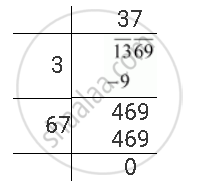Share

# Find the Square Root of the Following Number by Division Method. 1369 - CBSE Class 8 - Mathematics

ConceptSquare Roots - Finding Square Root by Division Method

#### Question

Find the square root of the following number by division method.

1369

#### Solution

The square root of 1369 can be calculated as follows.∴ sqrt1369 = 37

Is there an error in this question or solution?

#### APPEARS IN

NCERT Solution for Mathematics Textbook for Class 8 (2018 to Current)
Chapter 6: Squares and Square Roots
Ex. 6.40 | Q: 1.06 | Page no. 107
Solution Find the Square Root of the Following Number by Division Method. 1369 Concept: Square Roots - Finding Square Root by Division Method.
S